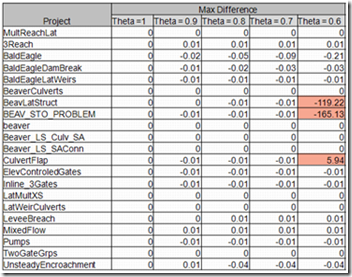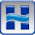## Monday, December 19, 2011

### Theta Implicit Weighting Factor and its Effect on Sample Datasets

Written by Aaron A. Lee   | WEST Consultants
Adding to the previous topic on the Theta Implicit Weighting Factor (Theta), this post takes an objective look at how the unsteady-flow option affects model output. Theta is a weighting factor for the spatial derivative used in solving the finite difference forms of the St. Venant equations. Adjusting Theta can improve model stability or increase the accuracy of the output. In a practical sense, how much is Theta really changing the solution? This post observes the influence of Theta by running the 21 installed (sample) projects in HEC-RAS version 4.1.

Theta can be found by navigating to Calculation Options and Tolerances under the Unsteady Flow Analysis Options menu. The default value is 1.0, but the user can define a value of Theta anywhere between 1.0 and 0.6. A value of 0.5 represents a half weighting explicit to the previous time step’s known solution, and a half weighting implicit to the current time step’s unknown. A value of 1.0 gives a fully implicit formula that is highly diffusive. In theory, a higher value will improve model stability but is less accurate in the solution. The opposite is true for lower values of Theta, which can make the model more sensitive to errors and lead to oscillations.

The table below summarizes the results for the sample projects included in the experiment. Water surface elevations (WSEL) are compared at each river station between the current plan and the default plan, Theta = 1.0. The values in the table are the largest maximum differences in WSEL for the entire reach. The cells in red are the plans that failed.Apart from the three crashed runs, the difference in the solutions is very small. The results demonstrate that for these simulations, the model is not highly sensitive to changes in Theta. Keep in mind that these models are relatively simple (shorter reach lengths, plain structures, uniform geometry) when compared to other unique project situations.

Changing Theta has a direct effect on how the solution is solved, but other factors may have more of an effect on stability and accuracy. The Hydraulic Reference Manual notes that factors such as cross-sectional properties, abrupt slope changes, flood wave characteristics, and complex hydraulic structures often overwhelm any stability considerations associated with Theta. When testing a model, pay special attention to the stability considerations listed above before laboring over Theta. While lowering Theta will yield (technically) more accurate results, it can also propagate errors where other factors may be causing problems. The User’s Manual suggests making sure that the computation interval is accurately defined, and that the maximum number of iterations is reasonable.

The HEC-RAS User’s Manual (page 8-32) suggests starting out with a Theta value of 1.0. Paraphrasing from the User’s Manual, page 8-32: “Once the model is up and running, the user should experiment with changing Theta towards a value of 0.6. If the model remains stable, then a value of 0.6 should be used. In many cases, there may not be an appreciable difference in the results when changing Theta from 1.0 to 0.6. However, every simulation is different, so you must experiment with your model to find the most appropriate value.”

The results of adjusting Theta for the 21 sample projects validates the approach suggested in page 8-32 of the HEC-RAS User’s Manual.

1.Very interesting post, thank you.

2.hello ,
3.